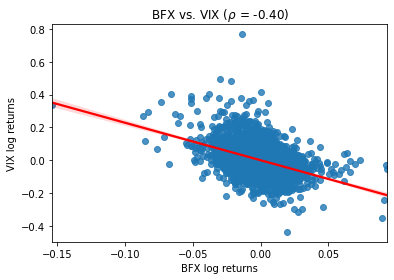# Euronext Brussels(BEL20) INDEX analysis in python

## Euronext Brussels(BEL20) INDEX is a European index. It consists of a minimum of 10 and a maximum of 20 companies.

Analyzing the Euronext Brussels BEL20 Index using Python involves collecting historical price data, performing data analysis, and creating visualizations to gain insights into the performance of the Belgian stock market. Here's a step-by-step guide on how to conduct BEL20 Index analysis in Python:

Import Libraries:
Start by importing the necessary Python libraries for data manipulation, analysis, and visualization. Commonly used libraries include pandas, numpy, matplotlib, and yfinance to fetch historical data:

Python
Copy code
import pandas as pd
import numpy as np
import matplotlib.pyplot as plt
import yfinance as yf
Data Retrieval:
Use the yfinance library or other financial data sources to fetch historical data for the BEL20 Index. Specify the start and end dates for the data you want to analyze:

Python
Copy code
Data Exploration:
Explore the fetched data to understand its structure and contents. Use functions like head(), tail(), describe(), and info() to inspect the dataset:

Python
Copy code
Data Visualization:
Create visualizations to analyze the historical performance of the BEL20 Index. Common visualizations include line charts to visualize index price movements:

Python
Copy code
plt.figure(figsize=(12, 6))
plt.title('BEL20 Index Price')
plt.xlabel('Date')
plt.ylabel('Price')
plt.legend()
plt.show()
Technical Analysis (Optional):
Perform technical analysis by calculating and visualizing technical indicators like moving averages, relative strength index (RSI), and MACD. Libraries like ta-lib can be used for these calculations.

Statistical Analysis (Optional):
Conduct statistical analysis to calculate summary statistics, volatility measures, and correlations with other assets. numpy and pandas are useful for these calculations.

Sentiment Analysis (Optional):
Consider incorporating sentiment analysis of news articles or social media data related to the Belgian stock market to understand market sentiment's impact on the BEL20 Index.

Fundamental Analysis (Optional):
Analyze fundamental factors affecting the Belgian economy, such as GDP growth, inflation rates, and interest rates, which can influence the BEL20 Index's performance.

Prediction and Forecasting (Optional):
You can use time series forecasting techniques like ARIMA or machine learning models to make predictions about future BEL20 Index movements.

Risk Management and Decision Making:
Based on your analysis, formulate investment strategies, set risk management parameters, and make informed investment decisions regarding the BEL20 Index or Belgian stocks.

Keep your analysis up to date with the latest data to adapt to changing market conditions and make timely decisions.

Remember that investing in stock markets carries risks, and it's crucial to do thorough research, consider economic and geopolitical factors, and potentially consult with financial experts before making investment decisions based on your analysis of the BEL20 Index or any other stock index.
start='1985-01-01',
end='2021-08-13',
progress=False)

df[['simple_rtn','log_rtn']].tail(20)
def realized_volatility(x):
return np.sqrt(np.sum(x**2))
df_rv = df.groupby(pd.Grouper(freq='M')).apply(realized_volatility)
df_rv.rename(columns={'log_rtn''rv'}, inplace=True)
df_rv.rv = df_rv.rv * np.sqrt(12)
fig, ax = plt.subplots(21, sharex=True)
ax.plot(df)
ax.plot(df_rv)
[<matplotlib.lines.Line2D at 0x7f2509bbc510>,
<matplotlib.lines.Line2D at 0x7f2509c550d0>,
<matplotlib.lines.Line2D at 0x7f2509c552d0>]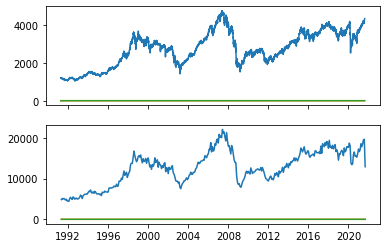fig, ax = plt.subplots(31, figsize=(2420), sharex=True)
ylabel = 'Stock price ($)') df.simple_rtn.plot(ax=ax) ax.set(ylabel = 'Simple returns (%)') df.log_rtn.plot(ax=ax) ax.set(xlabel = 'Date', ylabel = 'Log returns (%)') [Text(0, 0.5, 'Log returns (%)'), Text(0.5, 0, 'Date')]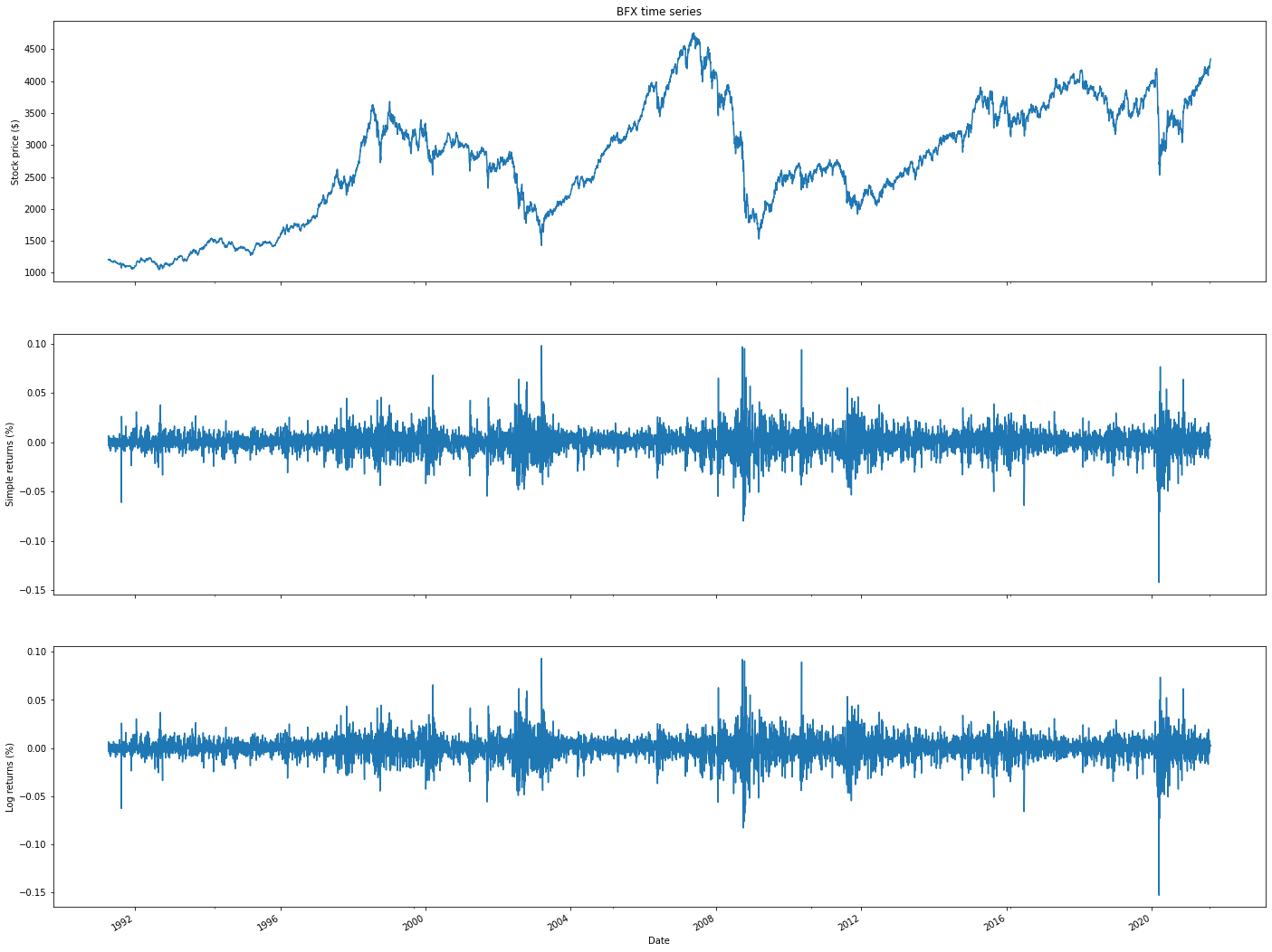import cufflinks as cf from plotly.offline import iplot, init_notebook_mode init_notebook_mode() df_rolling = df[['simple_rtn']].rolling(window=21) \ .agg(['mean''std']) df_rolling.columns = df_rolling.columns.droplevel() df_outliers = df.join(df_rolling) def indentify_outliers(rown_sigmas=3): x = row['simple_rtn'] mu = row['mean'] sigma = row['std'] if (x > mu + 3 * sigma) | (x < mu - 3 * sigma): return 1 else: return 0 df_outliers['outlier'] = df_outliers.apply(indentify_outliers, axis=1) outliers = df_outliers.loc[df_outliers['outlier'] == 1, ['simple_rtn']] fig, ax = plt.subplots() ax.plot(df_outliers.index, df_outliers.simple_rtn, color='blue', label='Normal') ax.scatter(outliers.index, outliers.simple_rtn, color='red', label='Anomaly') ax.set_title("BFX returns") ax.legend(loc='lower right') <matplotlib.legend.Legend at 0x7f2505f4a690>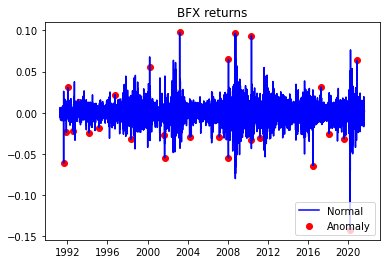r_range = np.linspace(min(df.log_rtn), max(df.log_rtn), num=1000) mu = df.log_rtn.mean() sigma = df.log_rtn.std() norm_pdf = scs.norm.pdf(r_range, loc=mu, scale=sigma) fig, ax = plt.subplots(12, figsize=(168)) # histogram sns.distplot(df.log_rtn, kde=False, norm_hist=True, ax=ax) ax.set_title('Distribution of BFX returns', fontsize=16) ax.plot(r_range, norm_pdf, 'g', lw=2, label=f'N({mu:.2f}{sigma**2:.4f})') ax.legend(loc='upper left'); df.log_rtn.plot(title='Daily BFX returns') <matplotlib.axes._subplots.AxesSubplot at 0x7f2505ec8a90>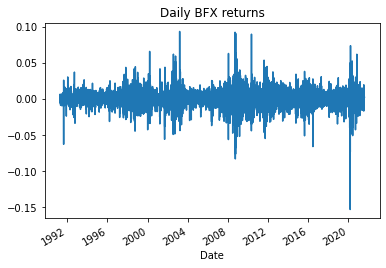df = yf.download(['^BFX''^VIX'], start='1985-01-01', end='2021-08-13', progress=False) df.tail() df = df[['Adj Close']] df.tail() df.columns = df.columns.droplevel(0) df = df.rename(columns={'^BFX''bfx''^VIX''vix'}) df['log_rtn'] = np.log(df.bfx / df.bfx.shift(1)) df['vol_rtn'] = np.log(df.vix / df.vix.shift(1)) df.dropna(how='any', axis=0, inplace=True) corr_coeff = df.log_rtn.corr(df.vol_rtn) ax = sns.regplot(x='log_rtn', y='vol_rtn', data=df, line_kws={'color''red'}) ax.set(title=f'BFX vs. VIX ($\\rho$= {corr_coeff:.2f})', ylabel='VIX log returns', xlabel='BFX log returns') [Text(0, 0.5, 'VIX log returns'), Text(0.5, 0, 'BFX log returns'), Text(0.5, 1.0, 'BFX vs. VIX ($\\rho\$ = -0.40)')]